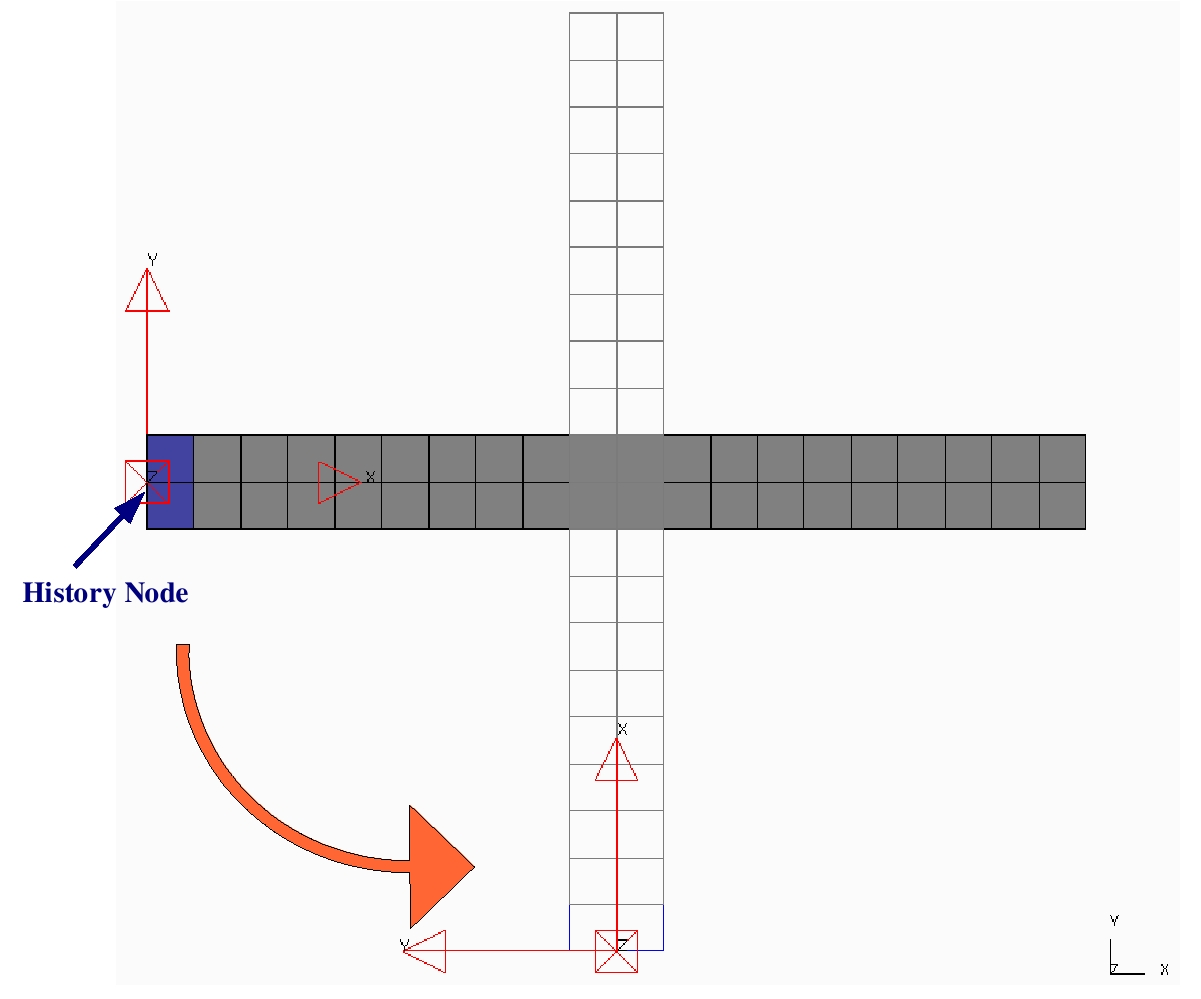# How can nodal accelerations be evaluated in LS-DYNA?

Within in the consider example, a rigid body (20mm x 200mm; 2 x 20 elements) rotates around his centre of mass, cf. Figure 1.
The initial rotational velocity is wz=628,32 rad/s,  which means that the  history node rotates within 10ms 360° around the z-axis.

Figure 1: Sketch of the considered initial boundary value problem.For a closer look on the `IACCOP` option in `*CONTROL_OUTPUT` (average of nodal acceleration), two elements (blue) are modeled as elastic material, so that in this part oscillations are initiated due to not exactly defined initial conditions (centripetal acceleration is missing).

## Analytical solution

The two components of the nodal acceleration can be computed analytically in the following way.

#### Tangential acceleration in local y-direction:

• tangential velocity:

vy = w * r = 628,32 rad/s * 100 mm = 62832 mm/s.

• tangential acceleration:

ay = dvy / dt = 0, because w = const.

#### Radial acceleration in local x-direction:

• No motion of the measuring point with respect to the center of rotation. Thus, .
• The centripetal force is given due to the rotary motion via

Fx = m * w2 * r.

• Using F = m * a, this results in the following expression:

ax = w2 * r = (628,32 rad/s)2 * 100 mm = 39478602 mm/s².

## Options concerning acceleration output in LS-DYNA

`• IACCOP (*CONTROL_OUTPUT)     Flag to average or filter nodal accelerations output to file “NODOUT” and      the time history database “D3THDT”:           EQ.0:  no average (default),           EQ.1:  averaged between output intervals`
`• REF (*DATABASE_HISTORY_NODE_LOCAL)     Output reference:           EQ.0: Output is in the local system fixed for all time from the                beginning of the calculation.           EQ.1: Output is in the local system which is defined by the                *DEFINE_COORDINATE_NODES. The local system can change                orientation depending on the movement of the three defining nodes.                The defining nodes can belong to either deformable or rigid parts.           EQ.2: Output is relative to the local system which is defined by the                *DEFINE_COORDINATE_NODES option. The local system can                change orientation depending on the movement of the three defining                nodes. If dynamic relaxation is used, the reference location is reset                when convergence is achieved.`
`• INTOPT (*ELEMENT_SEATBELT_ACCELEROMETER)     Integration option. If the accelerometer undergoes rigid body translation      without rotation this option has no effect; however, if rotation occurs,      option 1 may provide better agreement with the output of the      accelerometer.           EQ.0: velocities are integrated from the global accelerations and                 transformed into the local system of the accelerometer          EQ.1: velocities are integrated directly from the local accelerations                 of the accelerometer.`

#### *DATABASE_HISTORY_NODE, IACCOP=0

The acceleration as well as the velocity oscillate periodically, because the output is given with respect to the fixed, global coordinate system. The derivative of the velocity corresponds to the direct acceleration output and provides a kind of prefiltering. The extremal values reflect the analytical solution.

#### *DATABASE_HISTORY_NODE, IACCOP=1

The acceleration output has, in this example, the same prefiltering effect than using the derivative of the velocity.

#### *DATABASE_HISTORY_NODE_LOCAL, IACCOP=0, REF=0

This yields the same result than using `*DATABASE_HISTORY_NODE`, `IACCOP=0`, because the local coordinate system corresponds to the global one.

#### *DATABASE_HISTORY_NODE_LOCAL, IACCOP=0, REF=1

The nodal output, i. e., the absolute acceleration and velocity, is given within the defined free movable, local coordinate system. The y-accelerations obtained on the hand from the derivative of the velocity and on the other hand directly from the database are, apart from the prefiltering effect, identical. The velocity is constant and the acceleration is zero. One obtaines the analytical solution for the centripetal acceleration  in x-direction only from the LS-DYNA database and not from the derivative of the x-velocity.

#### *DATABASE_HISTORY_NODE_LOCAL, IACCOP=0, REF=2

In this case, the relative velocity and relative acceleration are given with respect to the free movable, local coordinate system in the database output. As there is no motion of the node relative to the defined coordinate system, the (relative) velocity as well as the (relative) acceleration output is zero.

#### *ELEMENT_SEATBELT_ACCELEROMETER, IACCOP=0, INTOPT=0

The output of the nodal values correspond to the values obtained using `*DATABASE_HISTORY_NODE_LOCAL`, `IACCOP=0`, `REF=1`. Here, no oscillations occur, because, the output node belongs to the rigid body. Beside these oscillations, the results are equal to the results obtained using `*DATABASE_HISTORY_NODE_LOCAL`.

#### *ELEMENT_SEATBELT_ACCELEROMETER, IACCOP=0, INTOPT=1

Using option `INTOPT=1`, the velocity of the node is computed based on the local instead on the global acceleration. Thus, the centripetal acceleration is given via the derivative of this velocity. However, the linear increase of this velocity is not physical and should not be used for other evaluations.

dk 09/09